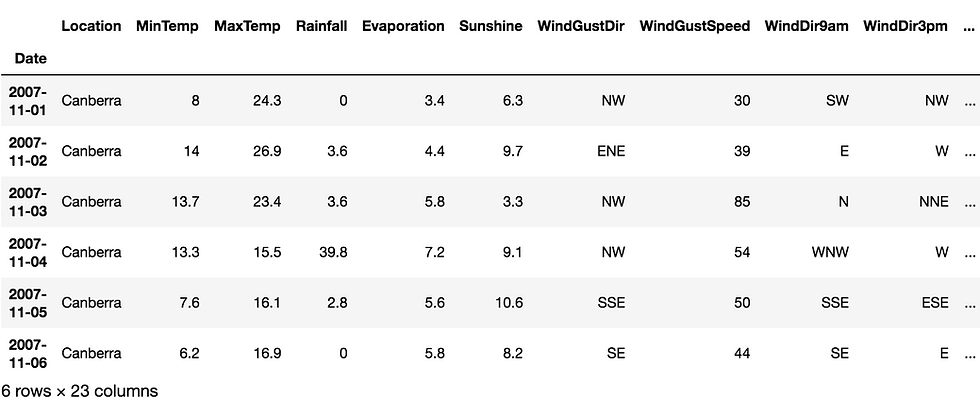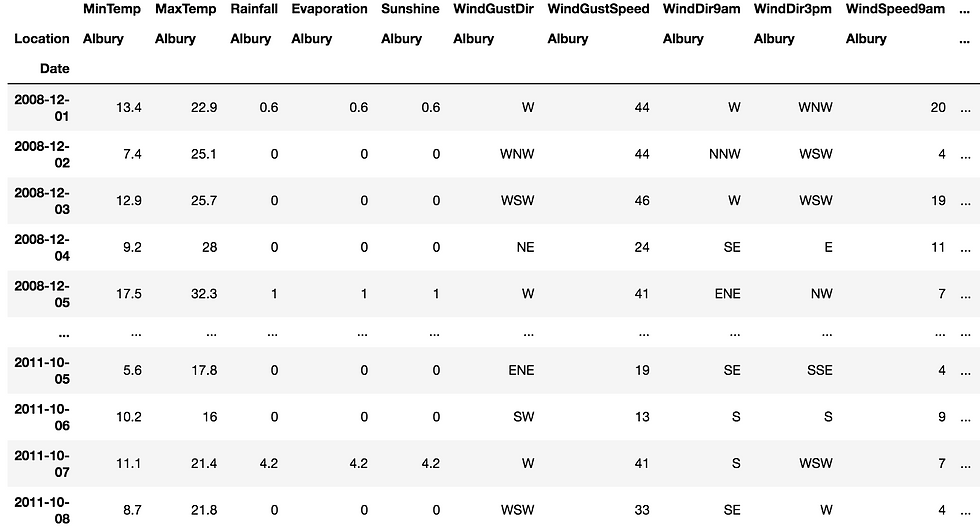top of pageSearch

# Pandas For Data Science

Data Science

Data Science is a blend of various tools, algorithms, and machine learning principles with the goal to discover hidden patterns from the raw data. Data science is the field of study that combines domain expertise, programming skills, and knowledge of mathematics and statistics to extract meaningful insights from data. Data science practitioners apply machine learning algorithms to numbers, text, images, video, audio, and more to produce artificial intelligence (AI) systems to perform tasks that ordinarily require human intelligence. In turn, these systems generate insights which analysts and business users can translate into tangible business value.

Machine Learning

Machine Learning (ML) is a branch of Data Science,It can be defined as giving the ability of decision making to machines without being explicitly trained by humans (which is Unsupervised Learning).It contains all the necessary algorithms for training machines to become better at decision making. ML requires data to train the machines.This data pattern are found out and better results are provided by the machine.Machine learning is a set of algorithms that predict an outcome given a set of input variables. Data Science is the discipline that builds, programs, runs and feeds these machine learning algorithms.

List of Common Machine Learning Algorithms

Here is the list of commonly used machine learning algorithms. These algorithms can be applied to almost any data problem:

1. Linear Regression

2. Logistic Regression

3. Decision Tree

4. SVM

5. Naive Bayes

6. kNN

7. K-Means

8. Random Forest

9. Dimensionality Reduction Algorithms

11. GBM

12. XGBoost

13. LightGBM

14. CatBoost

We are going to see Python Libraries for Date Science:

#### NUMPY

NumPy (Numerical Python) is a library for the Python programming language, adding support for large, multi-dimensional arrays and matrices, along with a large collection of high-level mathematical functions to operate on these arrays, is a perfect tool for scientific computing and performing basic and advanced array operations.The library offers many handy features performing operations on n-arrays and matrices in Python. It helps to process arrays that store values of the same data type and makes performing math operations on arrays easier.In my previous blog, you can find out Numpy basics and functions . Click here to read the blog.

PANDAS

Pandas is a library created to help developers work with “labeled” and “relational” data intuitively. It’s based on two main data structures: “Series” (one-dimensional, like a list of items) and “Data Frames” (two-dimensional, like a table with multiple columns). Pandas allows converting data structures to DataFrame objects, handling missing data, and adding/deleting columns from DataFrame, imputing missing files, and plotting data with histogram or plot box. It’s a must-have for data wrangling, manipulation, and visualization.

```#import libraries
import numpy as np
import pandas as pd

```

```#series data

data=pd.Series(("A","B","C","D"))
data

```

output:

```0    A
1    B
2    C
3    D
dtype: object

```

```#read the weather dataset from kaggle

d

``````#remove NaN values
df=d.fillna(method="ffill",axis="columns")
df``````#print particular rows values using date index
df["2007-11-01":"2007-11-06"]
df``````
#compress the level in dataframe column
stacked=df.stack()
stacked``````#Convert dataframe tabular data to Numpy array
df.to_numpy()``````#groupby  particular data
g=df.groupby('Location')
g``````h=g.get_group('Albury')
h``````#plotting
%matplotlib inline
h.plot()``````#pivot allows you to transform or reshape data
df.pivot(index='Date',columns='Location')

```Conclusion

In Above we see about what is Data Science ,Machine Learning ,and worked with datasets using pandas.I Hope this will be useful.Thanks.

.......................